Thursday , July 18 2019

# Mensuration

Learn how to measure the Area and Perimeter of 2D figures and Surface Area and Volume of 3D figures.

### Square: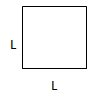Area:  2 × Length

Perimeter:  4(Length) or ( L+L+L+L )

### Rectangle:

Area:  L×B

Perimeter:  2(L+B) or (L+L+B+B)

### Triangle:Area: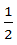× Base × Height

Perimeter: Sum of all three sides.

### Circle:

Area:Circumference:### Semi Circle: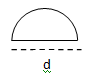Area: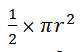Perimeter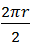### Parallelogram: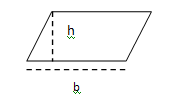Area: base × height

Perimeter: 2 (a+b) or Sum of all sides.

### Trapezium: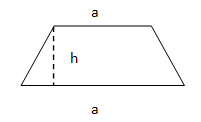Area:(a+b) h

Perimeter: Sum of all sides

### Cube: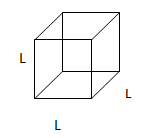Surface Area: 6L2

Volume: L3 or L × L × L

### Cuboid: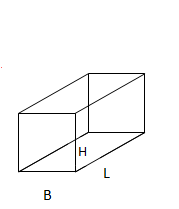Surface Area: 2 (BL+BH+LH)

Volume: L × B × H

### Cylinder: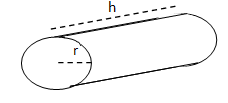### Prism: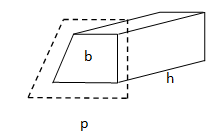Surface Area: (Perimeter of the base area (p) + 2 (base area))

Volume: Base area × height

### Sphere: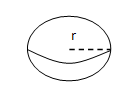Surface Area: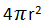Volume: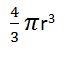For Hemisphere:

Surface area: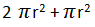Volume: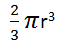## Properties Of A Circle

Circle Definitions: Geometrical Properties of Circle: If 2 chords in a circle area congruent, then …

### One comment

1.